# Simple harmonic motion Questions and Answers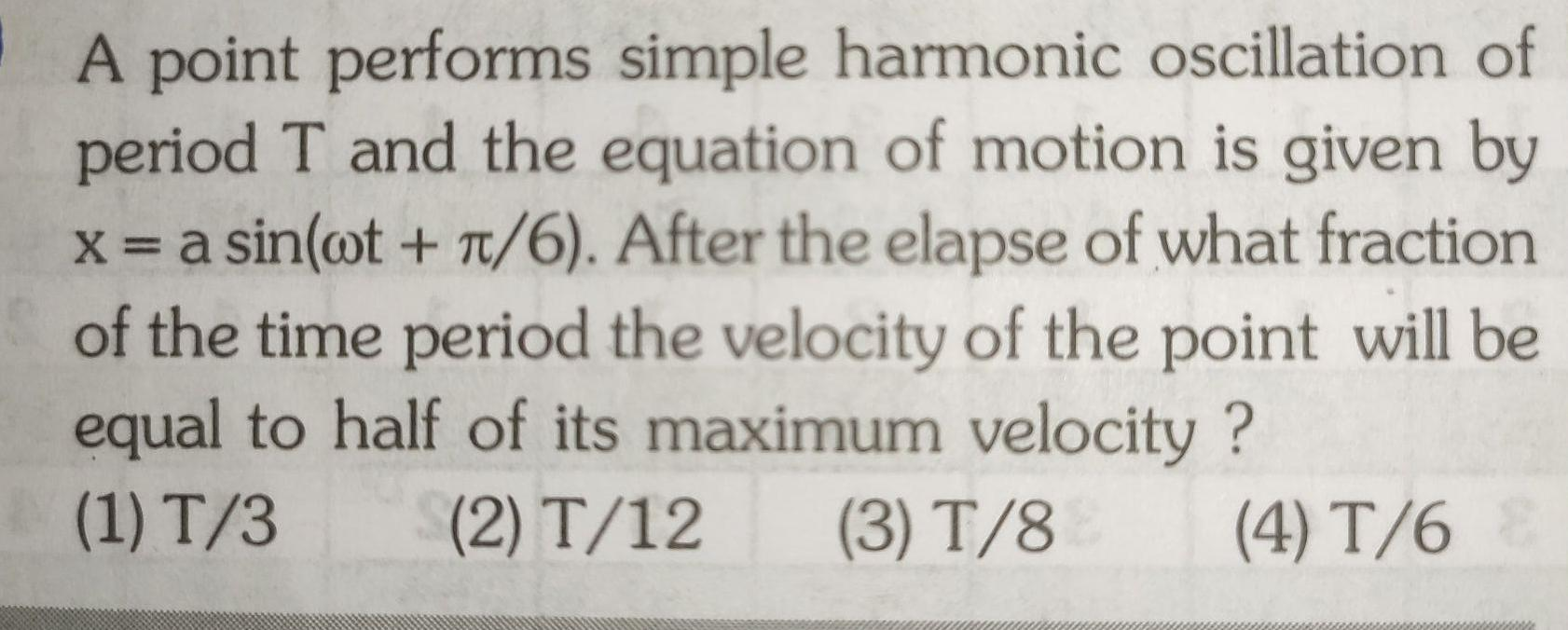Physics
Simple harmonic motion
A point performs simple harmonic oscillation of period T and the equation of motion is given by x a sin wt 6 After the elapse of what fraction of the time period the velocity of the point will be equal to half of its maximum velocity 1 T 3 2 T 12 3 T 8 4 T 6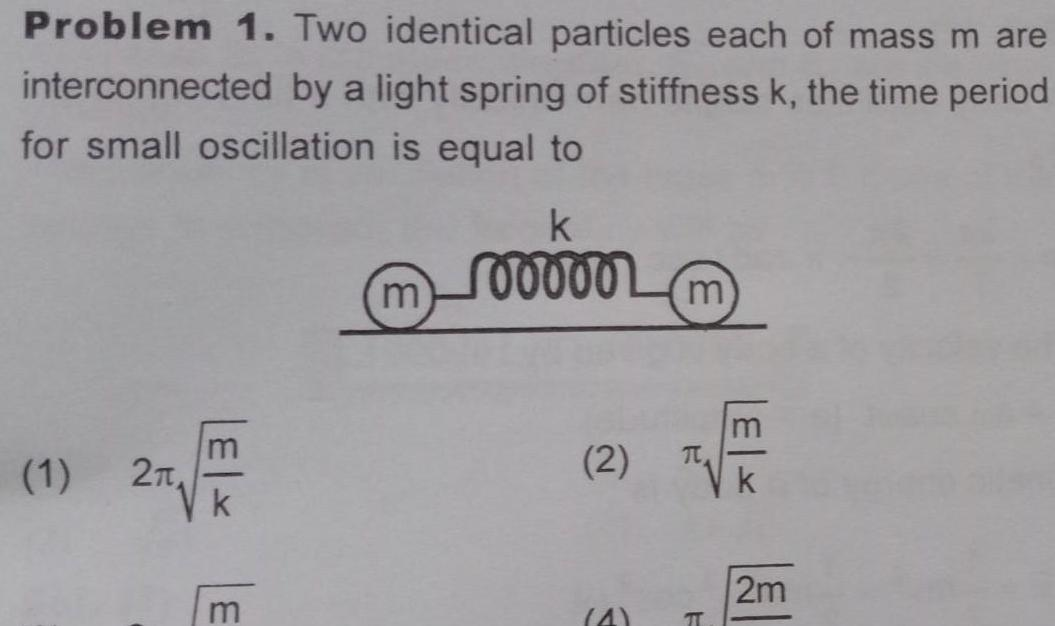Physics
Simple harmonic motion
Problem 1 Two identical particles each of mass m are interconnected by a light spring of stiffness k the time period for small oscillation is equal to 1 2 m k 100000 2 4 m F k 2m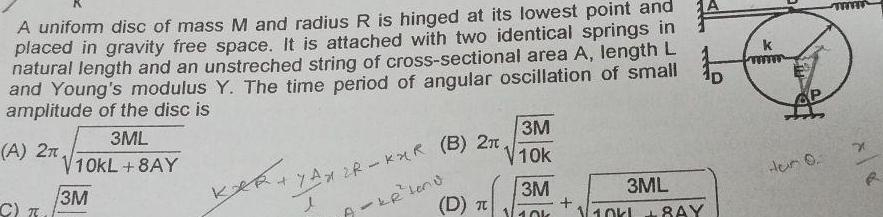Physics
Simple harmonic motion
A uniform disc of mass M and radius R is hinged at its lowest point and placed in gravity free space It is attached with two identical springs in natural length and an unstreched string of cross sectional area A length L and Young s modulus Y The time period of angular oscillation of small amplitude of the disc is 3ML A 2n V10KL 8AY 3M C T 17AX 2R KXR B 2n l A KR Leno kehi 3M V10k D T 3M 40k 3ML 8AY k www AP Hun 0 m x R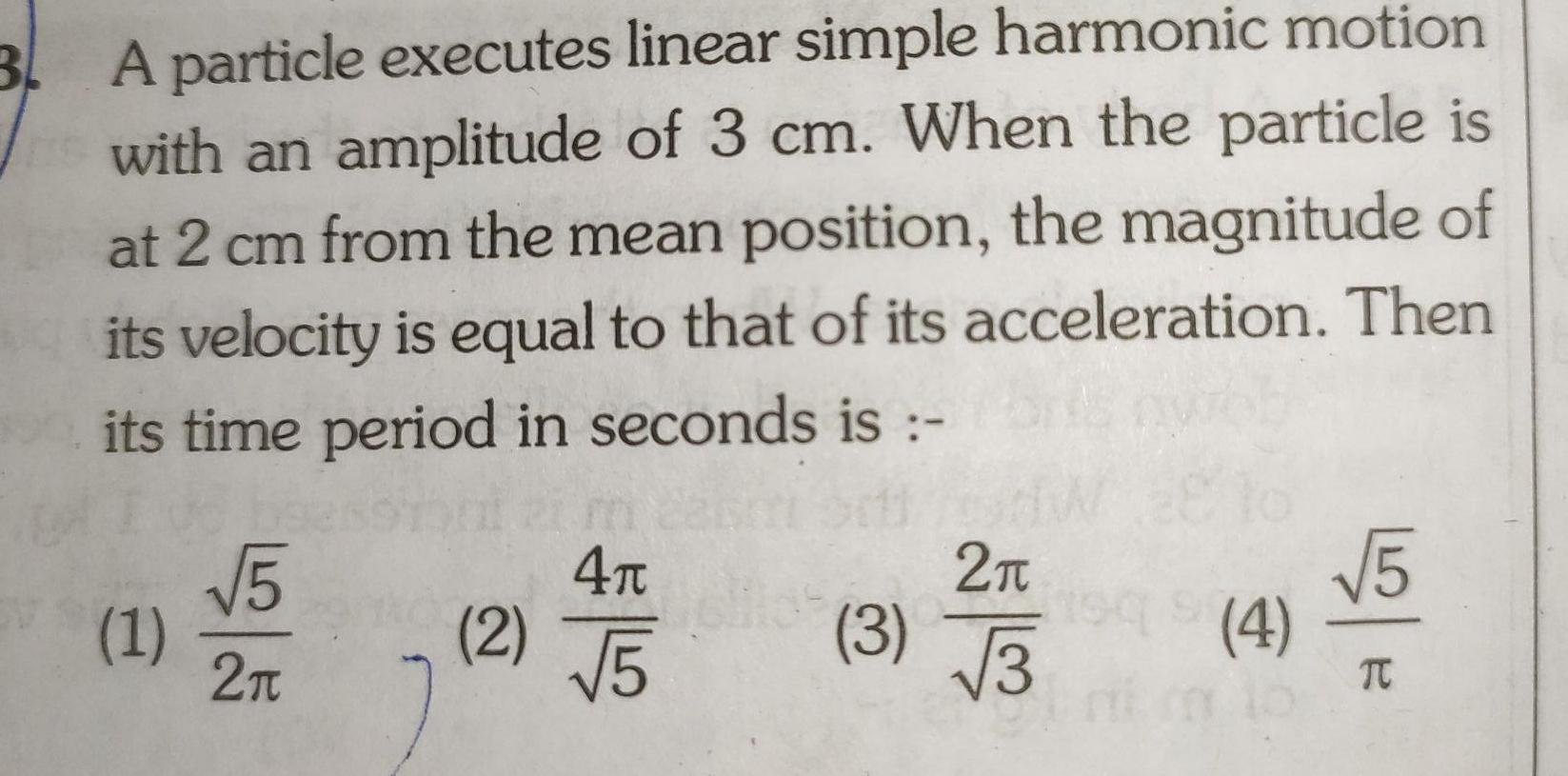Physics
Simple harmonic motion
3 A particle executes linear simple harmonic motion with an amplitude of 3 cm When the particle is at 2 cm from the mean position the magnitude of its velocity is equal to that of its acceleration Then its time period in seconds is 1 5 2 2 4 5 3 2 3 vo 1949 4 5 TC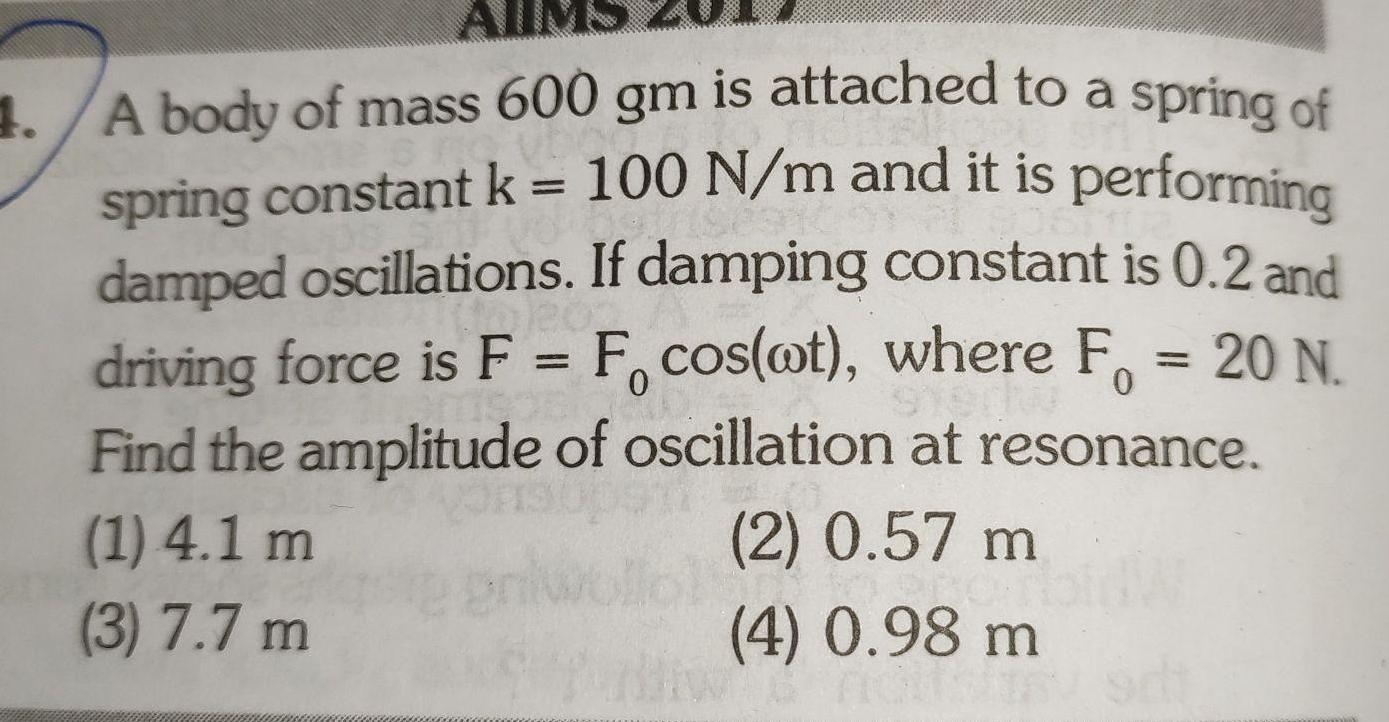Physics
Simple harmonic motion
AllM 1 A body of mass 600 gm is attached to a spring of spring constant k 100 N m and it is performing damped oscillations If damping constant is 0 2 and driving force is F F cos ot where F 20 N Find the amplitude of oscillation at resonance 0 2 0 57 m 4 0 98 m 1 4 1 m 3 7 7 m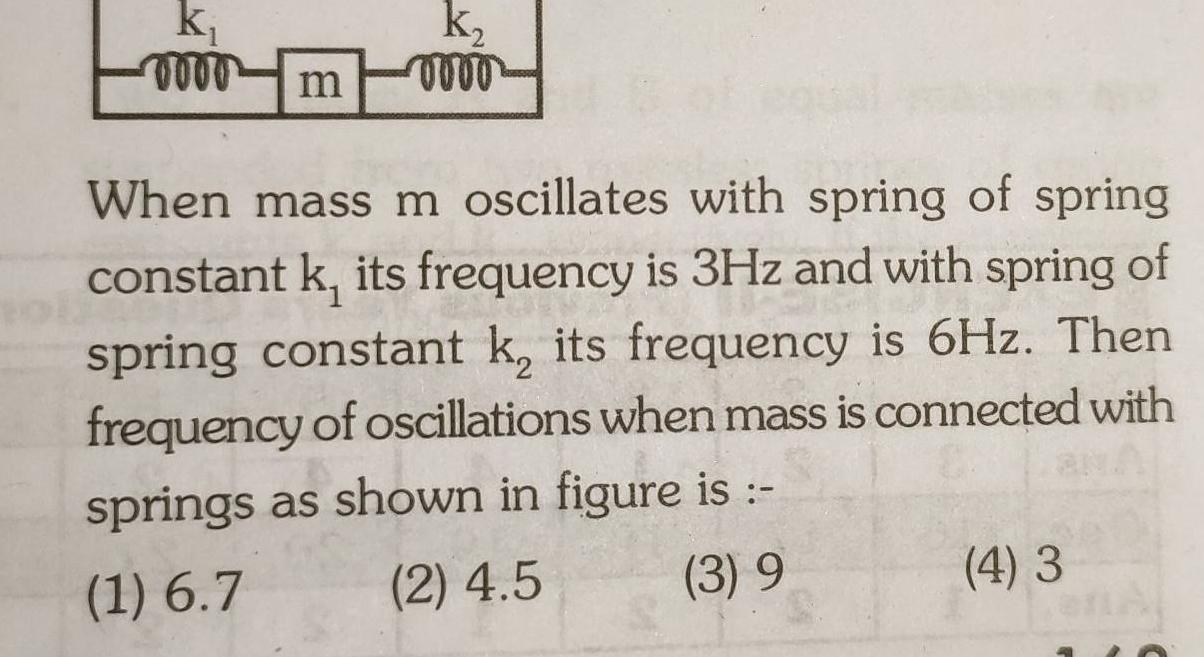Physics
Simple harmonic motion
k m k When mass m oscillates with spring of spring constant k its frequency is 3Hz and with spring of spring constant k its frequency is 6Hz Then frequency of oscillations when mass is connected with springs as shown in figure is 1 6 7 2 4 5 3 9 4 3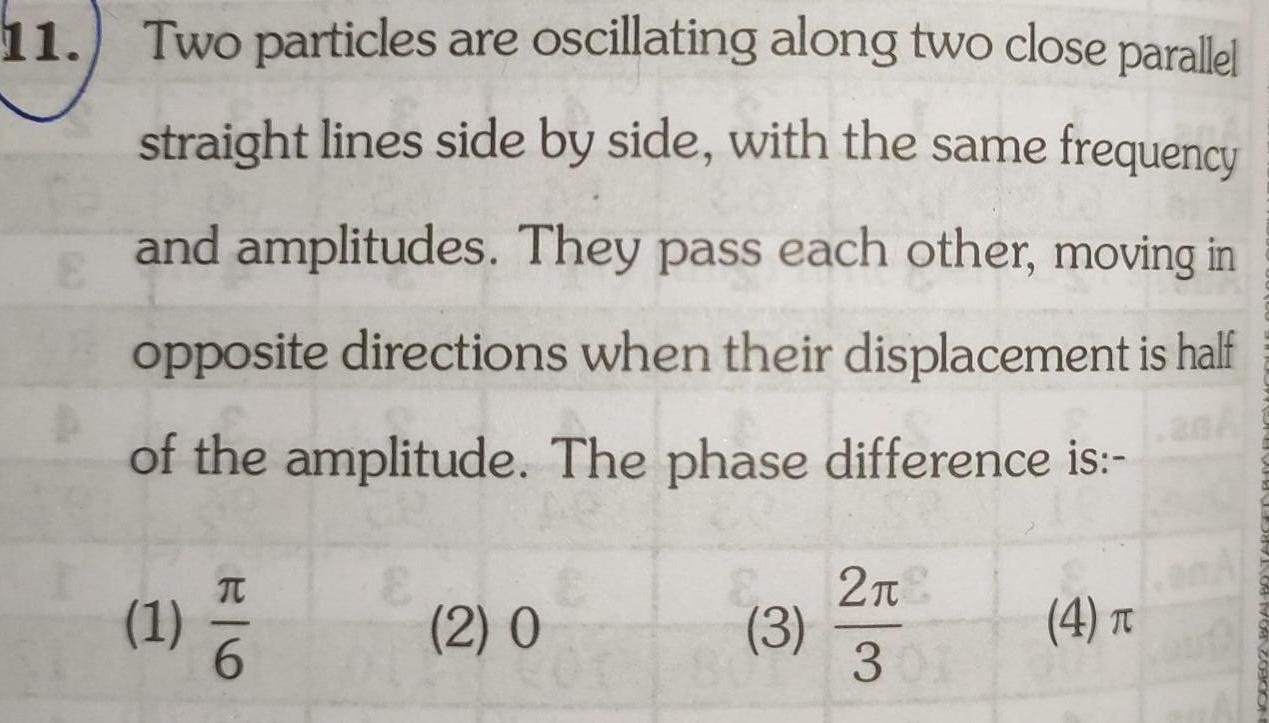Physics
Simple harmonic motion
11 Two particles are oscillating along two close parallel straight lines side by side with the same frequency and amplitudes They pass each other moving in opposite directions when their displacement is half E of the amplitude The phase difference is 1 26 2 0 3 2 301 4 T RENK M 2000ot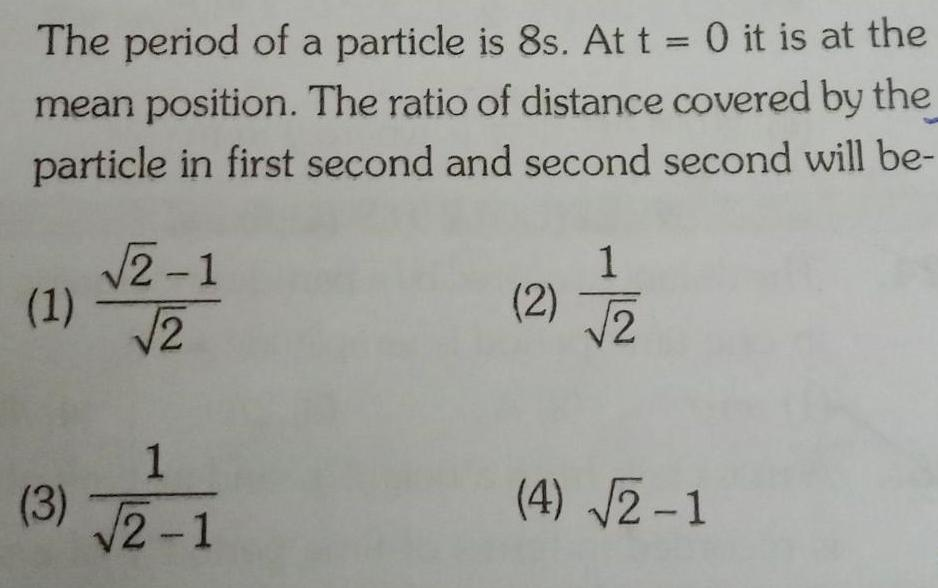Physics
Simple harmonic motion
The period of a particle is 8s At t 0 it is at the mean position The ratio of distance covered by the particle in first second and second second will be 1 3 2 1 2 1 2 1 2 1 2 4 2 1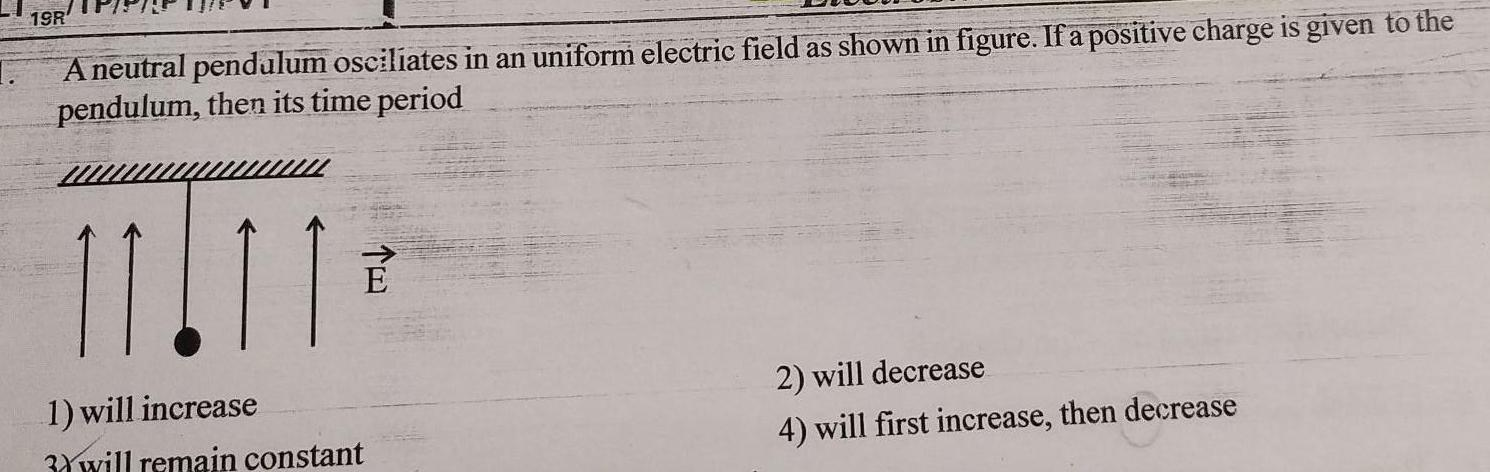Physics
Simple harmonic motion
19R A neutral pendulum osciliates in an uniform electric field as shown in figure If a positive charge is given to the pendulum then its time period 11 11 1 will increase 3 will remain constant 2 will decrease 4 will first increase then decrease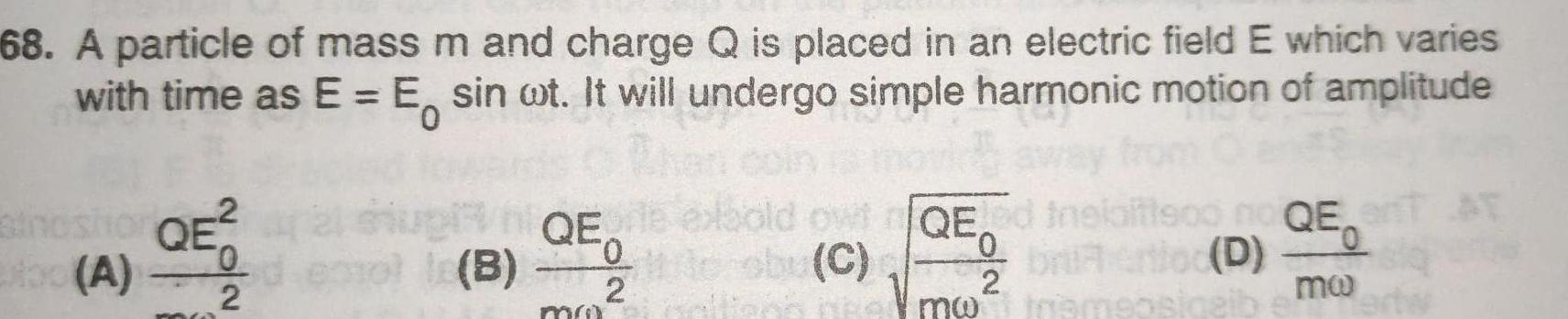Physics
Simple harmonic motion
68 A particle of mass m and charge Q is placed in an electric field E which varies with time as E E sin ot It will undergo simple harmonic motion of amplitude 2 thoshor QE 0 xbo A 2 le B QE 0 2 mu bold owtQE ed sby C me 0 Vmw bria ertloc D motramsosiqeib QET AT mo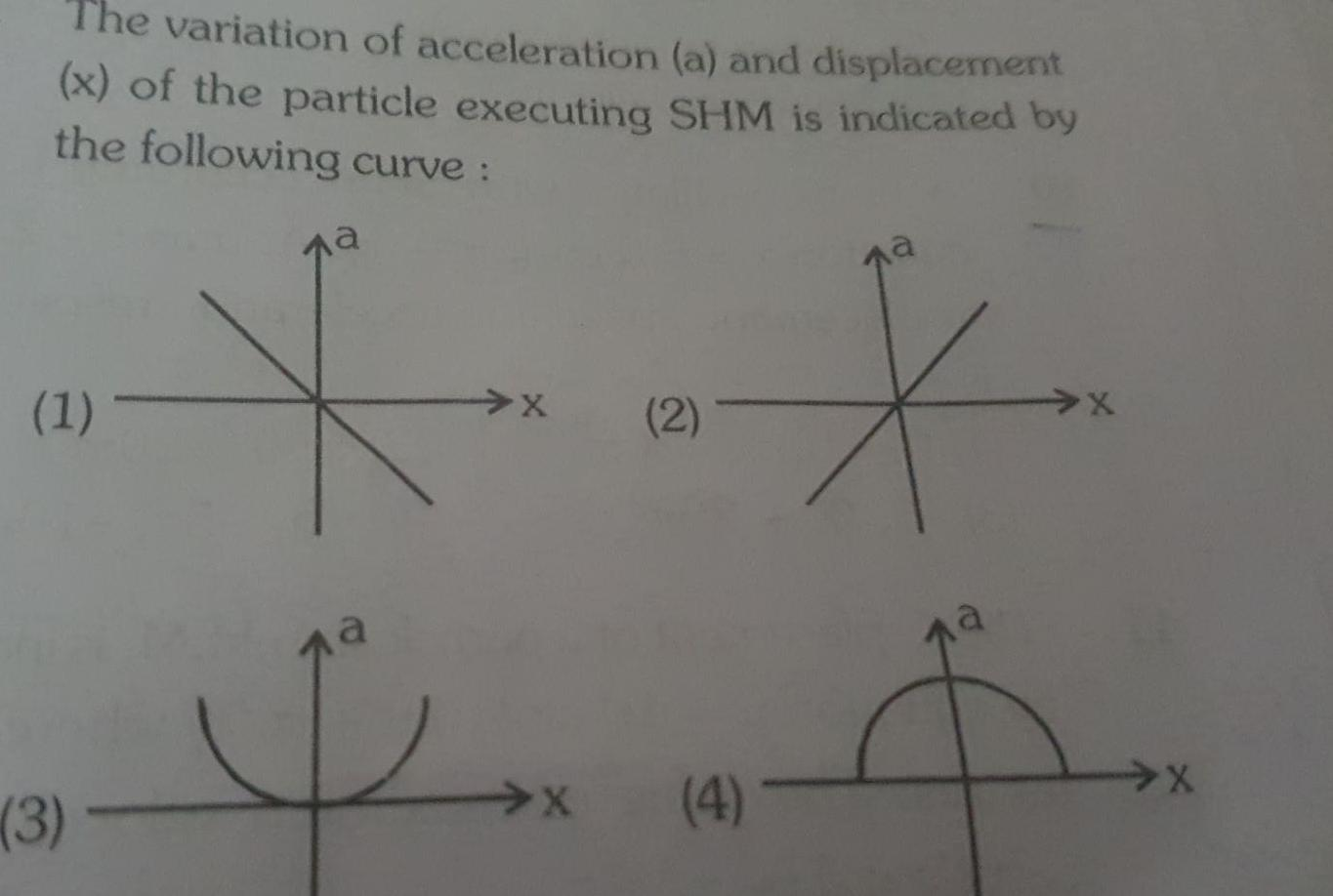Physics
Simple harmonic motion
The variation of acceleration a and displacement x of the particle executing SHM is indicated by the following curve 1 3 na X 2 X LA X 4 X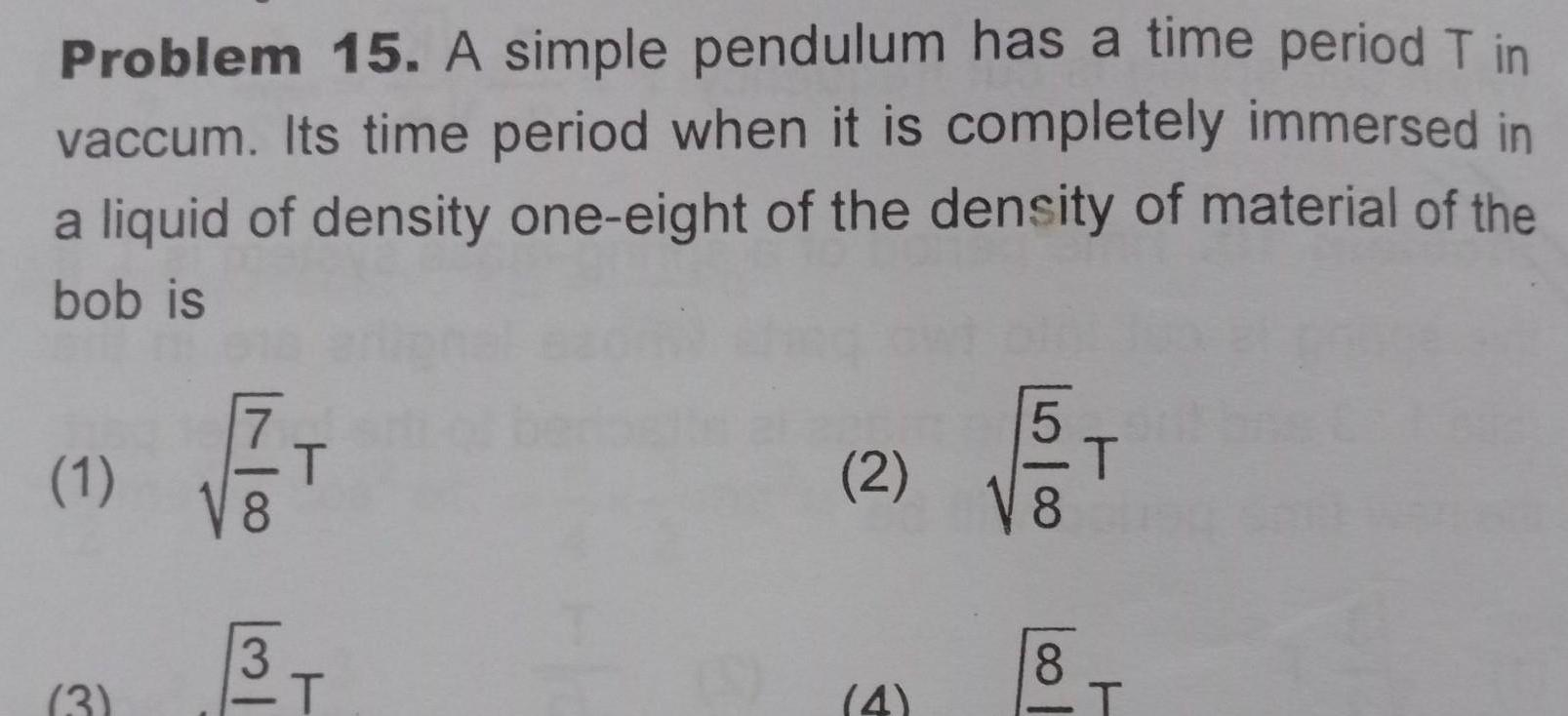Physics
Simple harmonic motion
Problem 15 A simple pendulum has a time period T in vaccum Its time period when it is completely immersed in a liquid of density one eight of the density of material of the bob is 1 3 T 3 T 2 4 8 8 T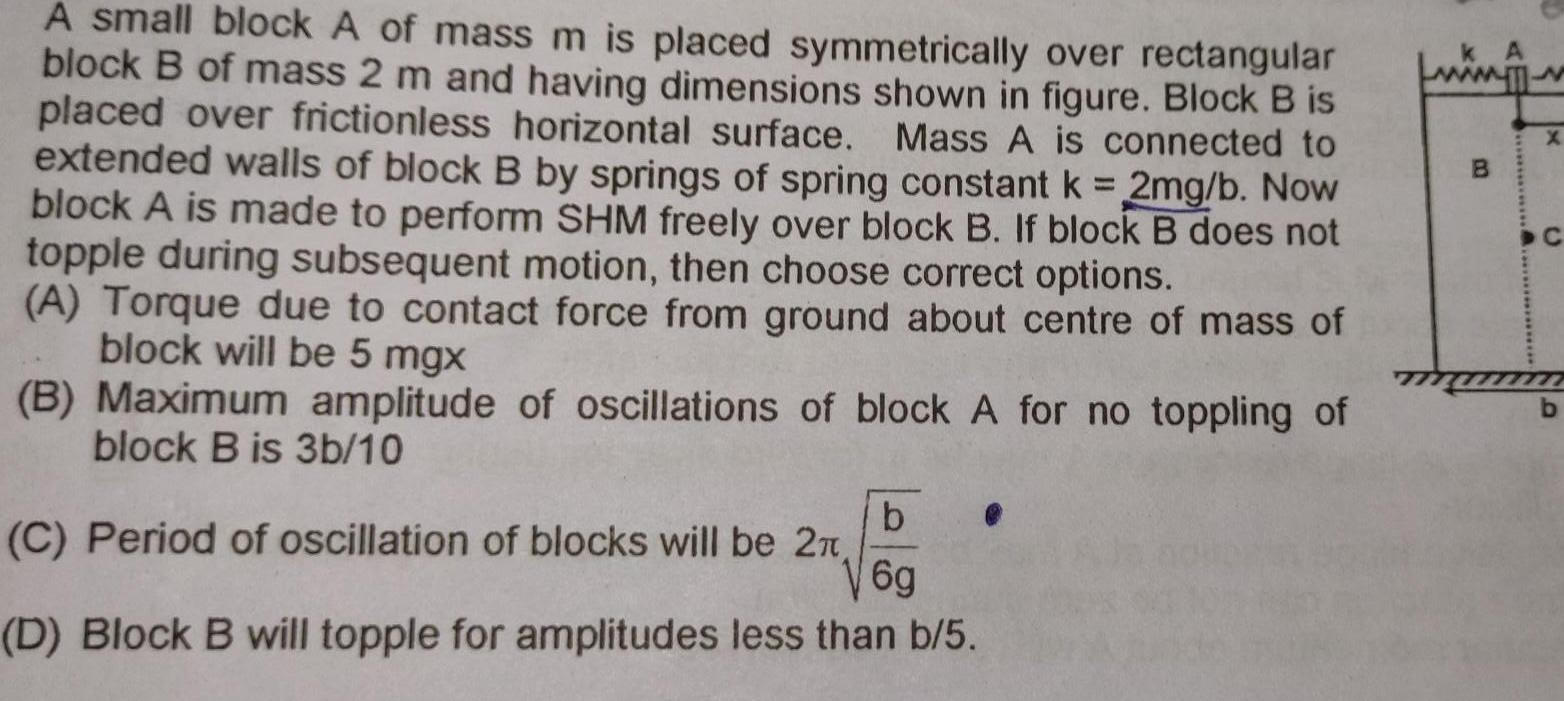Physics
Simple harmonic motion
A small block A of mass m is placed symmetrically over rectangular block B of mass 2 m and having dimensions shown in figure Block B is placed over frictionless horizontal surface Mass A is connected to extended walls of block B by springs of spring constant k 2mg b Now block A is made to perform SHM freely over block B If block B does not topple during subsequent motion then choose correct options A Torque due to contact force from ground about centre of mass of block will be 5 mgx B Maximum amplitude of oscillations of block A for no toppling of block B is 3b 10 b V6g D Block B will topple for amplitudes less than b 5 C Period of oscillation of blocks will be 2 B AT X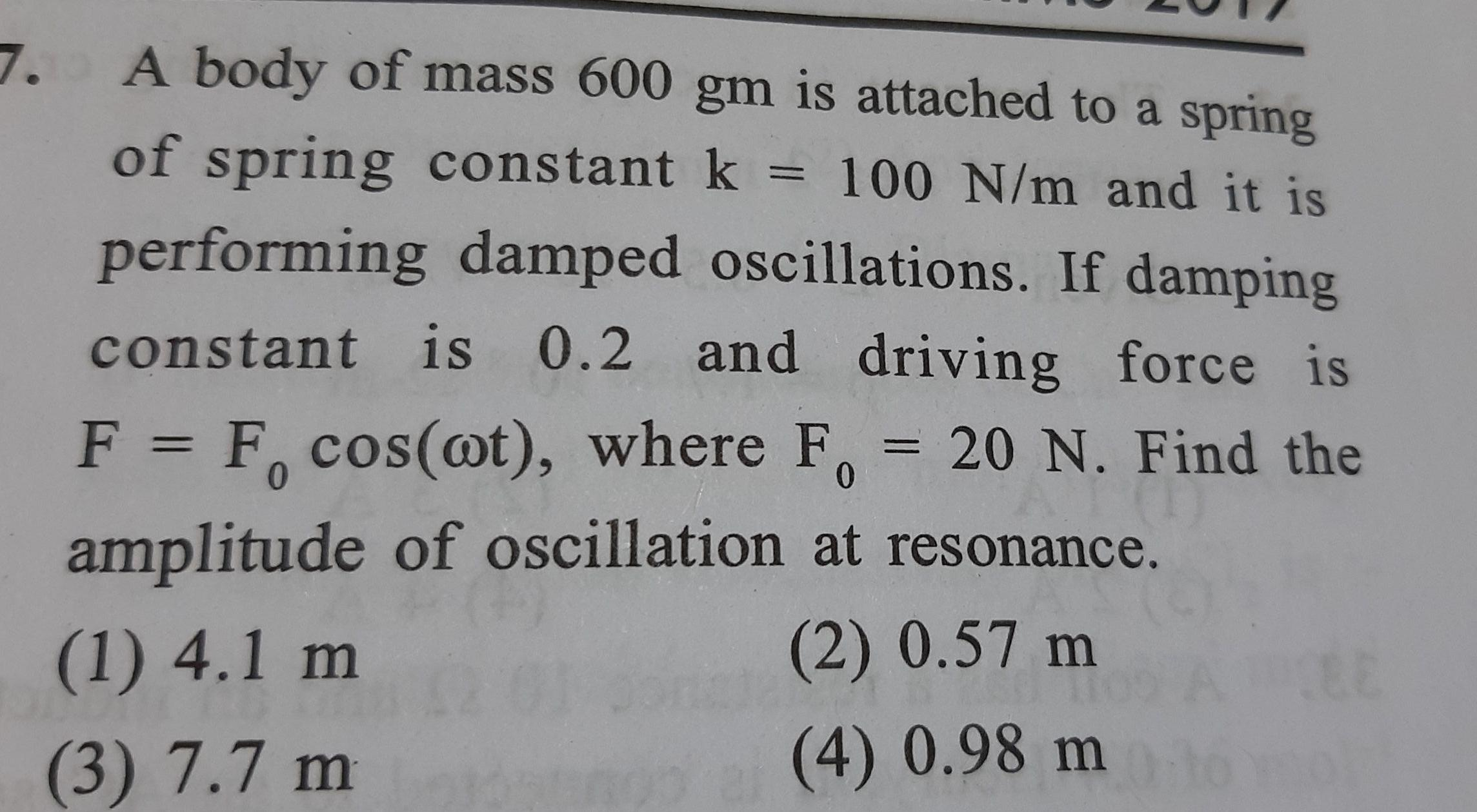Physics
Simple harmonic motion
7 A body of mass 600 gm is attached to a spring of spring constant k 100 N m and it is performing damped oscillations If damping constant is 0 2 and driving force is F 1 F cos ot where F 20 N Find the amplitude of oscillation at resonance 0 1 4 1 m 2 0 57 m 3 7 7 m 4 0 98 m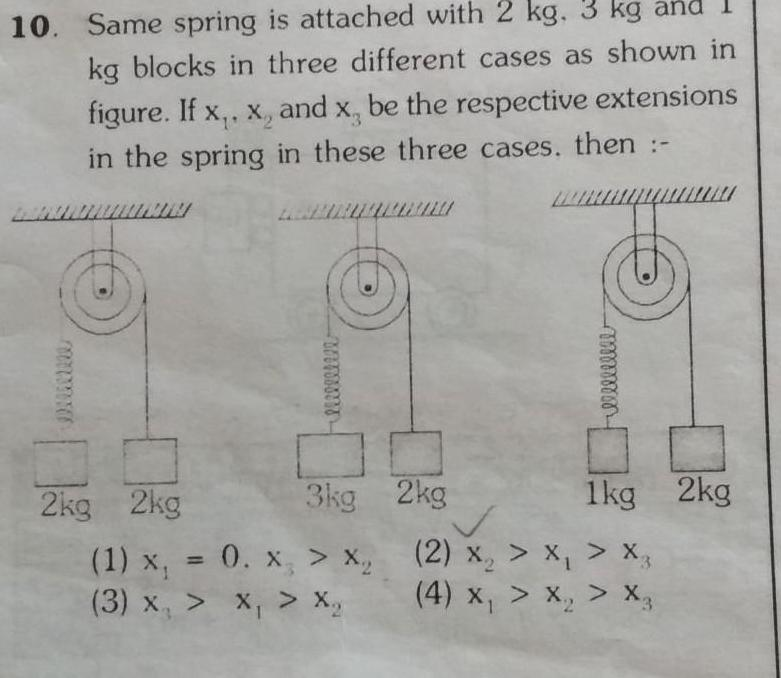Physics
Simple harmonic motion
10 Same spring is attached with 2 kg 3 kg and kg blocks in three different cases as shown in figure If x x and x be the respective extensions in the spring in these three cases then www lilllleee 2kg 2kg 3kg 1 x 0 x X 3 x X Xq Felllllllll 2kg 1kg 2kg 2 x x Xz x X 4 X X X3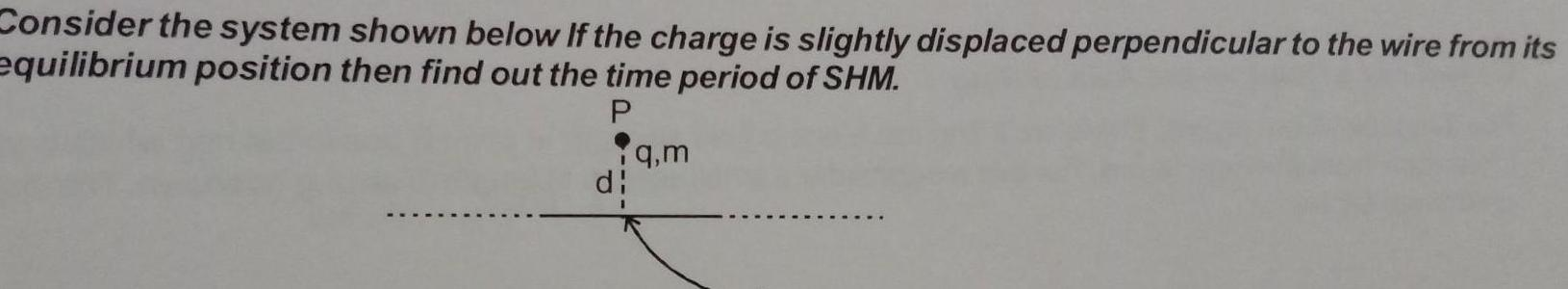Physics
Simple harmonic motion
Consider the system shown below If the charge is slightly displaced perpendicular to the wire from its equilibrium position then find out the time period of SHM P di q m